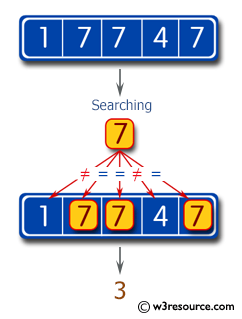﻿ Swift Basic Programming Exercise: Create a Swift program to count the number of 7's in a given array of integers - w3resource# Swift Basic Programming Exercise: Create a Swift program to count the number of 7's in a given array of integers

## Swift Basic Programming: Exercise-22 with Solution

Write a Swift program to create a Swift program to count the number of 7's in a given array of integers.

Pictorial Presentation:Sample Solution:

Swift Code:

``````func count7(_ input: [Int]) -> Int {
var ctr = 0

for num in input {
if num == 7 {
ctr += 1
}
}
return ctr
}
print(count7([1, 2, 7]))
print(count7([1, 7, 7]))
print(count7([1, 7, 7, 4, 7]))
```
```

Sample Output:

```1
2
3
```

Swift Programming Code Editor:

Improve this sample solution and post your code through Disqus

What is the difficulty level of this exercise?

﻿